### IMO Shortlist 2012 problem G6

Kvaliteta:
Avg: 4,5
Težina:
Avg: 8,0
Let$ABC$ be a triangle with circumcenter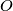$O$ and incenter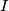$I$. The points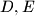$D,E$ and$F$ on the sides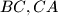$BC,CA$ and$AB$ respectively are such that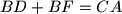$BD+BF=CA$ and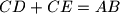$CD+CE=AB$. The circumcircles of the triangles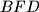$BFD$ and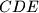$CDE$ intersect at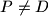$P \neq D$. Prove that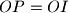$OP=OI$.
Izvor: Međunarodna matematička olimpijada, shortlist 2012